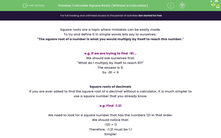# Calculate Square Roots (Without a Calculator)

In this worksheet, students will find square roots of whole numbers and decimals without using a calculator.Key stage:  KS 4

Year:  GCSE

GCSE Subjects:   Maths

GCSE Boards:   Pearson Edexcel, OCR, Eduqas, AQA,

Curriculum topic:   Number, Indices and Surds

Curriculum subtopic:   Structure and Calculation Powers and Roots

Popular topics:   Square Numbers worksheets

Difficulty level:#### Worksheet Overview

Square roots are a topic where mistakes can be easily made.

To try and define it in simple words lets say to ourselves:

"The square root of a number is what you would multiply by itself to reach this number."

e.g. If we are trying to find √81...

"What do I multiply by itself to reach 81?"

So √81 = 9

Square roots of decimals

If you are ever asked to find the square root of a decimal without a calculator, it is much simpler to use a square number that you already know.

e.g. Find √1.21

We need to look for a square number that has the numbers 121 in that order.

We should notice that:

√121 = 11

Therefore, √1.21 must be 1.1

Simple!

In this activity, you will find square roots of whole numbers and decimals without using a calculator.

When finding the square root of a decimal, you will need to relate it to a whole square number.

### What is EdPlace?

We're your National Curriculum aligned online education content provider helping each child succeed in English, maths and science from year 1 to GCSE. With an EdPlace account you’ll be able to track and measure progress, helping each child achieve their best. We build confidence and attainment by personalising each child’s learning at a level that suits them.

Get started